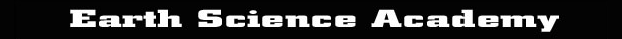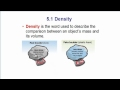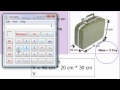[ ]    Teaching Videos: Density & Buoyancy - Chapter 5 // Earth Science YouTube Channel Playlist: ESA - Density6:59 Density: Basic Concepts (1) How density is a measure of its mass divided by its volume. (2) Determining density by measuring volume using a ruler or by water displacement12:07 Density: Word Problems (1) Using the density formula (D=m/v) (2) Using the volume formula (V=l*m*h) (3) Using the idea of density to solve a puzzle